# Learn Embedded Programming [Step by Step]

## Embedded Programming Tutorials for Beginners with Step By Step Guide.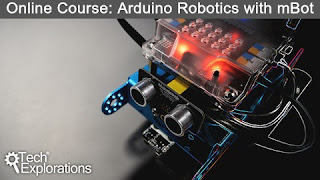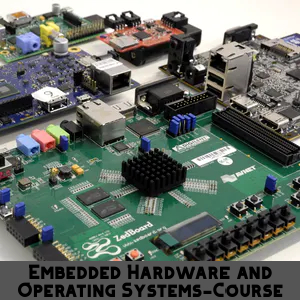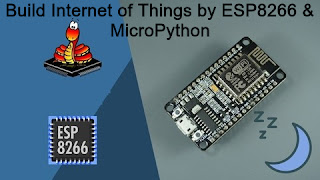## DC Motor's Speed Controlling Using PWM of Microcontroller

We can control the speed of a DC motor through a microcontroller. All we need to know about PWM .That means " Pulse Width Modulation ". It is a very popular technique and most important part of a microcontroller.  In this tutorial we will use PWM to create signal. By changing pulse width of a signal ,we can control the speed  .

## Basic Description:

At first we will connect a DC motor with PIC microcontroller and we will also connect two push buttons with it. One of those buttons will be used for increasing speed and another one for decreasing speed. That's all .

Now listen to me carefully, Just keep this in your mind
1. Increasing duty or Pulse Width will increase the speed of  DC motor .
2.Decreasing duty or Pulse Width will decreasing the speed of DC motor.

Now, we need to know about PWM [ Pulse Width Modulation ]

## Pulse Width Modulation (PWM)

We can create various signal through PWM. If we want to create 36KHz signal, that can be done by PWM .We can create any type signal. When we write program for creating signal through MCU, the CPP pin of microcontroller generate the signal according to the instructions. Lets consider, we have a 2Hz frequency signal. That means time period is 1/2=0.5 sec. This signal can be in various form. Look at the pictures :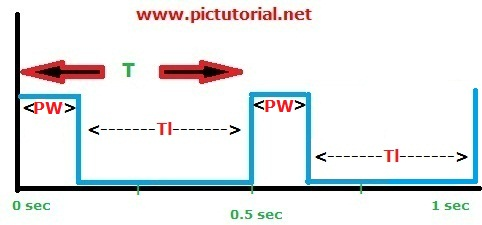Pulse width modulationwhen Set_Duty(127);PWM

Pic microcontroller's has two PWM or CPP module look at the picture .

In L293D's  EN1  pin we provide the signal. If you will provide highest pulse width, the speed will be highest. When you will decrease pulse width, the speed will also be decreased.
So if you don't know how to interface DC motor with microcontroller. It's recommended to read this tutorial ,

## DC Motor Interfacing with PIC Microcontroller in Proteus [step by step]

Time Period = Times of ON state + Times of OFF state .

### PWM has four functions

#### 4.PWM1_Stop();

Here most important thing is PWM1_Set_Duty(count) , If we increase the value of count, the speed increases. If we decrease the value of count, the speed decreases. Another important thing is the highest value of count is 255 and lowest is 0.

count proportional to PW
So, count = K * PW ----eq(1) where K = constant .

count/PW=K

When PW=T , count=255  and when PW=T/2 , count=127.5 or 127

255/T=127/(T/2) = K

and , inserting values of PW and count in eq(1)

255=K * T----eq(2)
127.5=K * (T/2)------eq(3)

Now eq(2)+eq(3) : 382.5= K { T +(T/2)}

or 382.5=K*(3T/2)
or 765=3*K*T
or T*K=255
or K=255/T
Now inserting value of  K into eq(1) , we get

Finally we get,  count =255*(PW/T), This is the relation between count, PW & T.
That was not very necessary for this tutorial. But I've shared all I knew.

When pulse width is equal to   T it means total time period it remains ON .  Now look at the picture given below :

Now create a project in proteus

## #MikroC Code :

`````` void main() {
unsigned int count=0;
CMCON=7;
TRISA.F0=1;
TRISA.F1=1;
TRISC.F1=0;
TRISC.F0=0;
PWM1_Init(1000);
PWM1_Start();
PORTC.F0=1;
PORTC.F1=0;
PWM1_set_duty(count);
while(1){
if(PORTA.F0==0){
delay_ms(100);
if(count>=255) {
count=255; }else{
count=count+15;
PWM1_set_duty(count);
}
}
if(PORTA.F1==0){
delay_ms(100);
if(count<=0){
count=0;  }else{
count=count-15;
PWM1_set_duty(count);
}
}
}
}

``````

## Thank You!

Ain't getting any visitors!

## Featured Post

### Rs 232 , Serial Communication with PIC Microcontroller in Proteus [step by step details]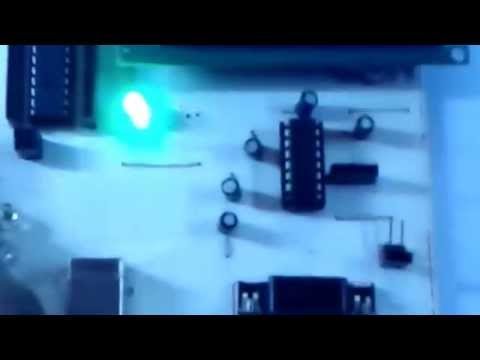## Tags

: (1) 18F2550 (1) 36KHz (3) and (1) arduino (1) Based (1) battery (1) Bipolar (1) Blinking (1) blinks (1) Bluetooth (1) button (1) circuit (1) clock (1) control (1) Db9 (1) DC Motor (2) digital (2) display (2) DS1307 (1) electronic (1) flash (1) flashing (1) HC-06 (1) home (1) how (1) How to (10) i2c tutorial (1) in (1) indicator (1) interface (8) interfacing (3) Interrupt (3) Introduction (1) IR Receiver (4) key pad (1) keyboard (1) keypad (1) lavel (1) Lcd 16x2 (2) lcd 2x16 (2) led (1) lm35 (2) LPG (1) machine (1) make (1) making (1) matrix (1) max232 (1) meter (2) microchip (4) microchips (3) mikroC (5) musical (1) NEC Protocol (4) pcb (5) PIC (3) pic controller (11) pic proteus (1) Pic Tutorial (12) pic18 (2) pic18f2550 (11) picRFモジュール (1) proteus (6) push (1) push button (1) PWM (1) real (1) Rs 232 (1) Rs232 (1) scroll (1) scrolling (1) Serial Port (1) simulation (2) step by step (7) step bystep (1) text (2) time (1) timer (4) timer0 (4) tone (1) tutorial (2) Unipolar (1) USB (1) usb 1.0 (1) USB HID (1) using (9) voltmeter (1) voting (1) with (2) work (1)

## Traffic Feed

Live Traffic Feed
Visitor Tracking